Color Mapping

Mapping an iteration count to a color in the Mandelbrot set

Method

When mapping the iteration count 1:1 to the color palette (linear mapping), the color distribution will be rather uneven because the "density" of color bands increases with decreasing distance to the Mandelbrot set. In order to obtain a more even distribution of the colors throughout the entire image, a non-linear function can be used to compress the colors at the lower end of the iteration count and stretch them at the higher end. To achieve this, the iteration count it is first normalized to a value x from 0 to 1, then the non-linear function is applied to x. The resulting value x which reaches from 0 to 1 as well is finally mapped to a color within the palette.

Example:

If the iteration count it in the image reaches from 50 to 200, then x is calculated as follows:

x = (it - minIt) / (maxIt - minIt)
= ([50...200] - 50) / (200 - 50)
= ([0...150]) / 150
= [0...1]

Next the non-linear function is applied to x:

x = f(x)

Finally x is mapped to a palette of 256 colors:

color = x * 255
= [0...1] * 255
= [0...255]

Comparison Chart

The following comparison chart shows different methods for mapping an iteration count to a color in the Mandelbrot set. Each image shows the same section of the Mandelbrot set, but with different linearities and – where applicable – different degrees (parameter n) for the color gradient. You can click on an image to open a JavaScript application in a new window / browser tab and draw the example image in full size (600 × 450 pixel). There you can alter the parameters by yourself to see the effect "live".

Mapping Method Example #1 Example #2 Example #3 Example #4 Example #5 Example #6 Example #7

Linear

This is the default color mapping with linear gradient.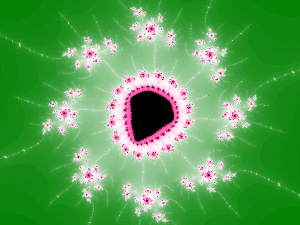¼ Sinus

This gradient corresponds to the first quarter of an sine wave.

Function:

x = sin(x*Pi/2)Auto-Logarithm

This function automatically adapts the degree of the gradient to the number of visible iterations/colors:

Function:

x = (log(it) - log(minIt)) / (log(maxIt) - log(minIt))

with it = iteration count, minIt = minimum iteration count and maxIt = maximum iteration count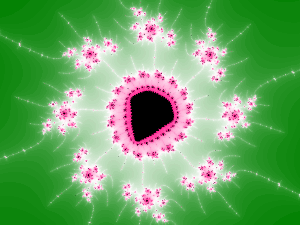Logarithm

This gradient has a logarithmic shape.

Function:

x = log(x*4n+1) / log(4n+1)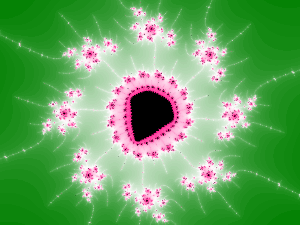n = 0.0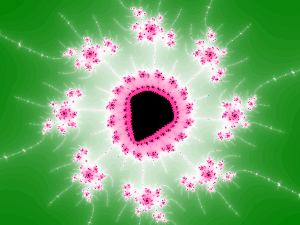n = 0.5n = 1.0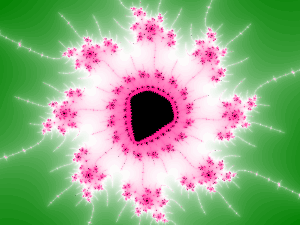n = 1.5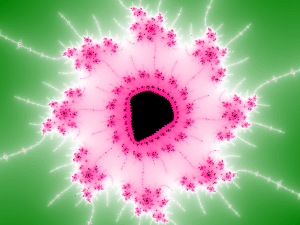n = 2.0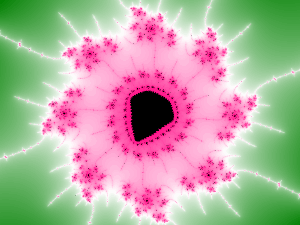n = 2.5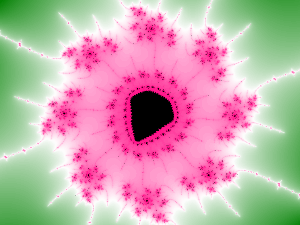n = 3.0

Gamma

Using a gamma function instead of a logarithmic one, the gradient becomes softer with less contrast.

Function:

x = x1/n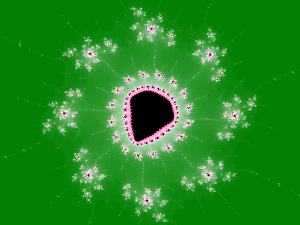n = 0.5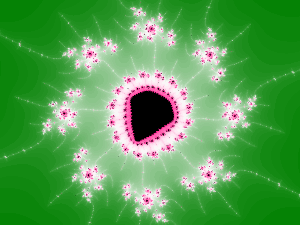n = 1.0n = 1.5n = 2.0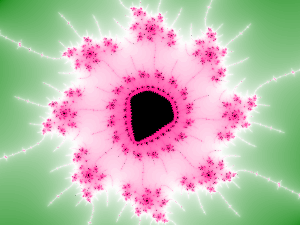n = 2.5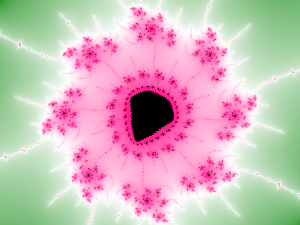n = 3.0n = 3.5

Hyperbolic Tangent

The hyperbolic tangent gradient curve is steeper than the logarithmic one and gives a higher contrast.

Function:

x = tanh(n*x) / tanh(n)n = 0.1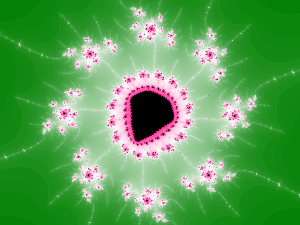n = 0.5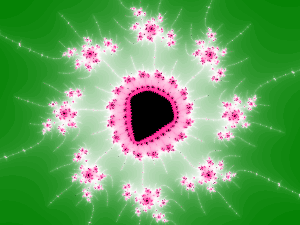n = 1.0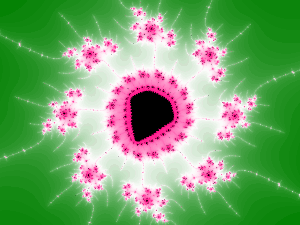n = 1.5n = 2.0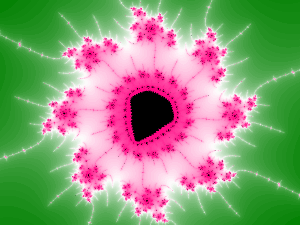n = 2.5n = 3.0

Palette Interpolation

In the above examples the normalized value x is mapped to a palette of 256 colors using linear interpolation:

color = x * 255
= [0...1] * 255
= [0...255]

In most cases the results are satisfying, but for high values of the parameter n some colors will appear unnaturally bright, resulting in a kind of "halo" around the Mandelbrot set. While this effect may be desirable, in some cases it can look ugly. To eliminate the "halo" effect, the junctions between the color gradients can be smoothed by using non-linear interpolation. An obvious way would be a Bézier curve, but this is a bit complicated to calculate since two additional control points are required which are difficult to define automatically. Much easier to implement is cosinus-shaped interpolation. For this, the palette points are connected by one half of a cosinus curve: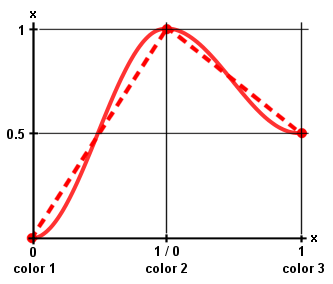Dotted line: Linear interpolation
Full line: (Co-)sinusoid interpolation

x = (cos(x * Pi + Pi) + 1) / 2;
or
x = (sin(x * Pi - Pi / 2) + 1) / 2;Example 1: Linear interpolation
Note the "halo" effect (yellow color) around the Mandelbrot set.Example 1: (Co-)sinusoid interpolation
The "halo" effect is gone.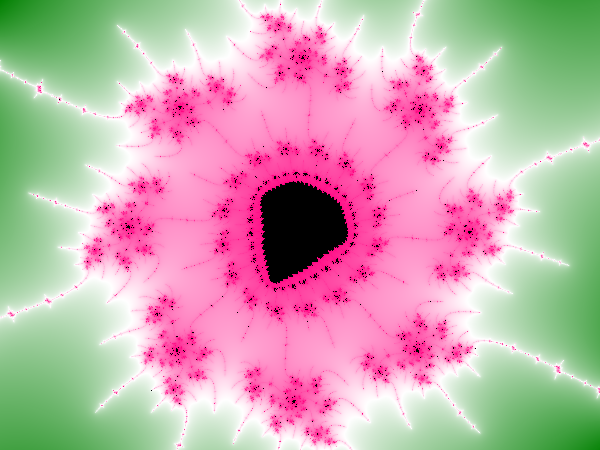Example 2: Linear interpolation
Note the "halo" effect (white color) between the green and the pink color.Example 2: (Co-)sinusoid interpolation
The white color is now smoother.

Homepage > Mandelbrot Set in JavaScript > Mathematics > Color Mapping